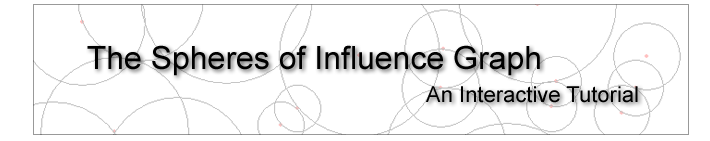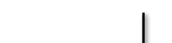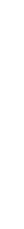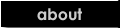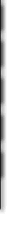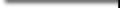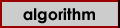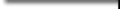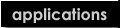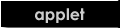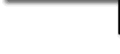# The Algorithm for computing the SIG in a plane

Input:A set of points in the plane.

• Take all the points in the plane, with no edges between any two points.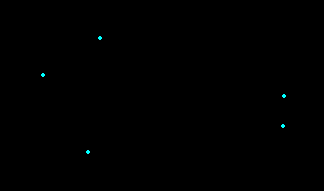• For each point in the plane, find the closest point to that point. If there are more than one closest point, arbitrarily choose any one of them. Compute the distance to that point. By distance, we will consider Cartesian distance in the plane.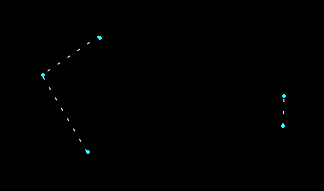• Draw a circle around each point, with a radius equal to the minimum distance computed above. This circle will pass through the closest point(s) of the center of the circle.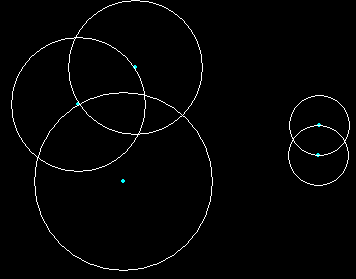• For every pair of points, connect them with an edge if and only if their circles intersect. In other words, if any two circles intersect, connect their centers with an edge.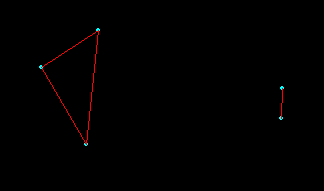Output:A connected or disconnected graph G. This is the Spheres-of-Influence Graph.

The animated image below demonstrates the above algorithm.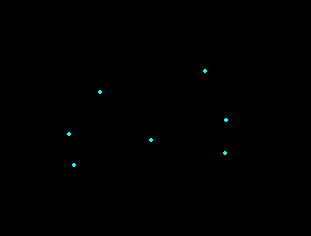The dotted lines indicate the shortest distance point from each of the individual points, Then the circles are drawn centered on the points and raidus equal to the distance to its nearest neighbor. The intersections of the circles are found and then the corresponding points are joined by an edge.

# Complexity Analysis

The SIG of a set of points can be found using the brute force approach as described above. There is a faster way of computing the SIG as well. The time requirements of both these techniques are discussed in some detail below.

1. Computing minimum distance using brute force approach takes O(n) time. This is done for each point, yielding O(n2) running time.
2. Finding intersections between circles can take O(n2) time, if the brute force approach is used. That is, by checking each circle against every other to see of they intersect. But a different approach yielding a O(nlogn) running time is possible by using an elegant line-segment intersection algorithm introduced by Bentley and Ottmann. This idea is explained in some detail.

# Finding Intersections

The Bentley and Ottmann algorithm finds the intersections between line segments by using a sweep line technique. A horizontal line L is swept on the set of line segments S. Let us assume that x= si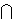sj be the intersection between two line segments, si and sj. Look at the figure below.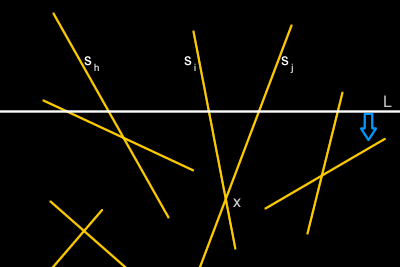Just before the sweepline L reaches the intersection point x, we can see that si and sj are adjacent on L. Therefore, it is only necessary to compute intersections between pairs of line segments that are adjacent on L. Of course, some of these adjacent lines do not actually intersect (for example sh and si) but that penalty is small enough to be insignificant in computing the total running time. Bentley and Ottmann showed that this algorithm can find intersections between pairs of line segments in O((n+k)logn) time, where k is the total number of intersections. Chazelle and Edelsbrunner reduced the running time to O(nlogn+k).

Now, how does this approach help us in finding intersections between circles? First, please note that the Bentley and Ottmann algorithm is based on the observation that a line segment is monotonic in both the X and Y directions on the plane. You, the astute reader will surely interject; that still does not help us with circles, since circles in a plane are not monotonic in the X or Y direction. And you will be right, but the trick is not to use the circles at all. Instead, we split the circles into four equal length arcs, as shown below.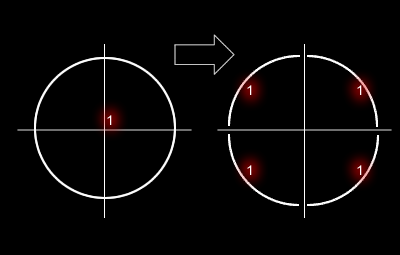Then we label each arc to indicate from which circle it comes from. Thus, each circle will have four arcs each. These arcs each are a quarter of the perimeter-length of the original circle. But most importantly, these are monotonic in both the X and Y directions. Which means, we can apply the Bentley and Ottmann algorithm to find intersections between them. The slight modification we have to make is this: when we find an intersection, we have to check whether or not they belong to the same circle (by checking the corresponding labels). If so, then we ignore the result. Otherwise we have an intersection between two circles. Therefore, this will yield an overall complexity of O(nlogn) for finding the intersection between circles. This approach was used by Hisham ElGindy to find the SIG in O(nlogn) time, as mentioned in .